# How To Prepare Magnetic Effects Of Current

###### BySafeer PP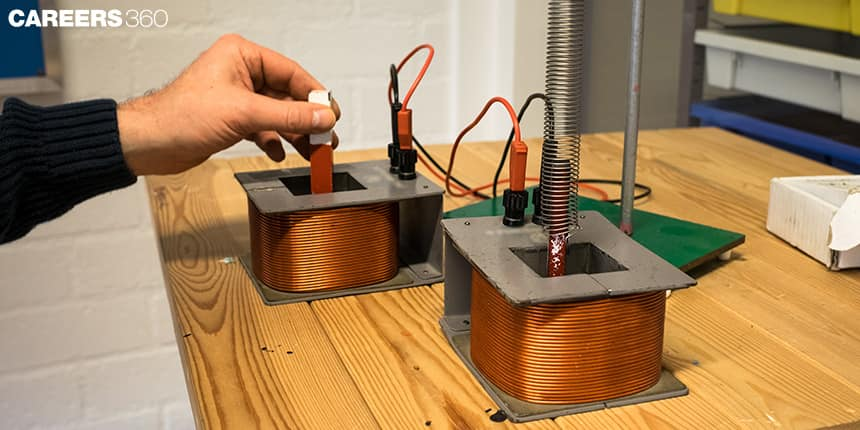###### Synopsis

Physics, chemistry and biology have equal weightage in the National Standard Examination in Junior Science (NSEJS). It is important to know how to prepare a chapter for NSEJS. Here are tips to help you prepare NSEJS for the chapter on Magnetic Effects Of Electric Current.###### Synopsis

Physics, chemistry and biology have equal weightage in the National Standard Examination in Junior Science (NSEJS). It is important to know how to prepare a chapter for NSEJS. Here are tips to help you prepare NSEJS for the chapter on Magnetic Effects Of Electric Current.

The National Standard Examination in Junior Science(NSEJS) is conducted every year across the country. Participation in the exam is not mandatory. Top students of NSEJS can participate in Indian National Olympiad For Junior Science(INJSO). The students preparing for NSEJS must be thorough with Class 9 and 10 NCERT science chapters. Here we share the tips to prepare one of the chapters in the NSEJS syllabus, which is Magnetic Effect Of Current that belongs to the Class 10 NCERT science book.

## Magnetic Effects Of Current: NSEJS Syllabus

Topics of the unit Magnetic Effect Of Current for NSEJS are listed below.

Magnetic field lines, Magnetic field due to current carrying conductor, field due to current carrying coil or solenoid; force on a current carrying conductor, Fleming’s left-hand rule and electric motor

Electromagnetic induction- emf and current induced, Fleming’s right-hand rule, electric generator; DC and AC current and domestic electric circuits.

Also Read| How To Prepare Sound For NSEJS

## Tips To Prepare Magnetic Effects Of Current For NSEJS

• Study and understand all the concepts from the NCERT book for Class 10.

• Understand how to apply the left-thumb rule and right-hand rule.

• Practice problems on finding the direction of force due to a current-carrying conductor in a uniform field.

• Solve questions to find the direction of the induced current.

• Understand Faraday's laws and Lenz’s laws well.

• Learn the working principle of electric motors and generators and understand their differences.

• Practice NSEJS previous year papers.

• Solve all the NCERT and NCERT exemplar problems on Magnetic Effects Of Electric Current.

Also Read| How To Prepare The Unit Light For NSEJS

## Points To Remember

• Magnetic field due to a current element at a point obeys inverse square law. That is, the field is inversely related to the square of the distance between the current element and the point.

• The direction of force due to a charge moving in a magnetic field of uniform strength is perpendicular to velocity and magnetic field.

• A conductor that carries a current placed in a uniform magnetic field experiences a force. This is the principle of the electric motor and the direction of force can be determined by Flemings' Left-Hand rule.

• If there is a relative motion between a uniform magnetic field and a conductor/coil an emf is induced in the conductor/coil. This is the working principle of the electric generator. The direction of the current due to the relative motion between a uniform magnetic field and a conductor can be determined by Fleming’s Right-Hand Rule.

• The AC supply voltage in India has a frequency of 50Hz, which is a period of 20 milliseconds.

• AC current changes both its magnitude and direction periodically.

## NSEJS Previous Year Questions On Magnetic Effects Of Current

Q-1- A bar magnet is allowed to fall freely from the same height towards a current carrying loop along its axis, as shown in the four situations I to IV. Arrows show the direction of conventional current. Choose the situations in which the potential energy of the magnet coil interaction is maximum (NSEJS 2018)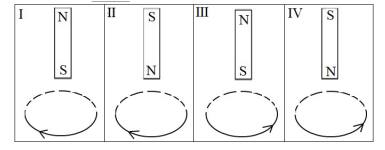(a) I, III (b) I, IV (c) II, IV (d) II, III

Q-2- When a charged particle with charge q and mass m enters a uniform magnetic field B with velocity v at right angles to B, the force on the moving particle is given by qvB. This force acts as the centripetal force making the charged particle go in a uniform circular motion with radius r =mv/qB. Now if a hydrogen ion and a deuterium ion enter the magnetic field with velocities in the ratio 2:1 respectively, then the ratio of their radii will be (NSEJS 2019)

(a) 1:2 (b) 2:1 (c) 1:4 (d) 1:1

Q-3- A conductor in the form of a circular loop carries a current I. The direction of the current is as shown. Then which figure represents the correct direction of magnetic field lines on the planes XY and XZ surfaces? (Consider those surfaces of the XY and XZ planes which are seen in the figure.-NSEJS 2019)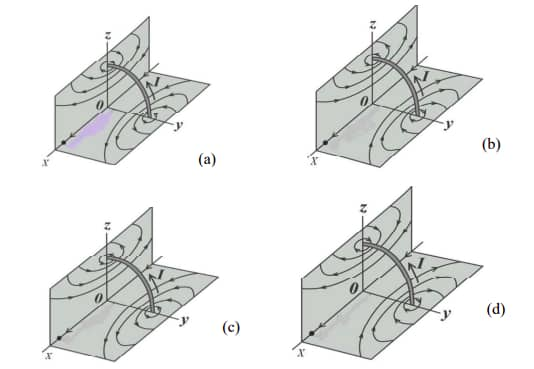Q-4- An infinitely long conductor when carrying current I, produces a magnetic field B around it. If such a conductor is placed along the X-axis, then the magnitude of ? at a distance r is given by the relation B = ?0 2?/4? ?, (where ?0/ 4? =10-7 NA-2 is a constant). The following figure shows such an infinitely long conductor placed along the X-axis carrying current I and B at S is 2 x 10-4 T, directed into the plane of the paper at S. Given r = 1 cm. Then, the correct statements are(NSEJS 2020)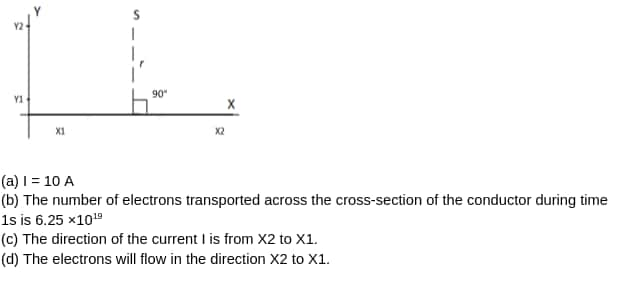Also Read| How To Prepare Work And Energy For NSEJS

## NSEJS Study Material For Magnetic Effects Of Current

• NSEJS Previous Year Papers

• NCERT Class 10 chapter Magnetic Effect Of electric Current

• Magnetic Effects Of Electric Current NCERT Exemplar questions

• Physical sciences
• Quantity
• Physics
• Electricity
• Electromagnetism
• Applied and interdisciplinary physics
• Physical quantities
• Electrical engineering
• Mechanics
• Metrology

## Careers360 helping shape your Career for a better tomorrow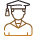#### 250M+

Students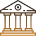#### 30,000+

Colleges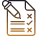#### 500+

Exams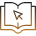E-Books